# Notes on machine learning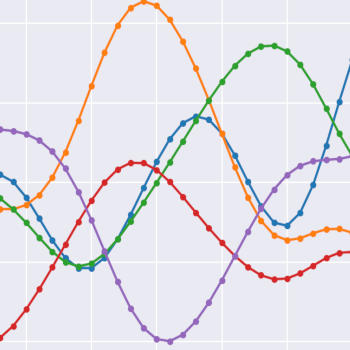## Gaussian processes

Blog series exploring Gaussian processes. Starts with building up an understanding of Gaussian procesess by implementing them from scratch in Python. Then goes to a practical example illustrating how to use a Gaussian process on a real-world problem using TensorFlow probability.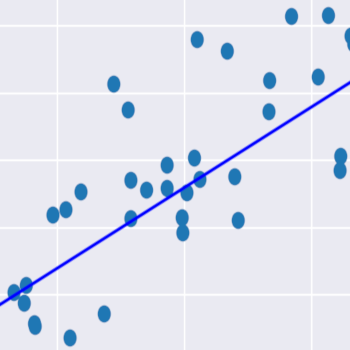## Regression quattro stagioni

This post will explore the foundation of linear regression and implement four different methods of training a regression model on linear data: simple linear regression, ordinary least squares (OLS), gradient descent, and markov chain monte carlo (MCMC).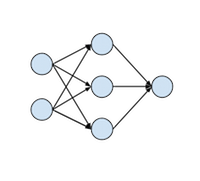## How to implement a neural network

Learn the basics of neural networks and how to implement them from scratch in Python. The tutorial starts with explaining gradient descent on the most basic models and goes along to explain hidden layers with non-linearities, backpropagation, and momentum. The math is explained along the way together with Python code examples.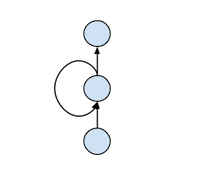## How to implement a RNN

Learn how to implement a recurrent neural network (RNN) in Python with the help of NumPy. The tutorial explains the basics of backpropagation-through-time and discusses some of the difficulties of training recurrent networks.## Multi-armed bandit implementation

Learn the basics of Bayesian multi-armed bandit models while implementing a simple example in Python.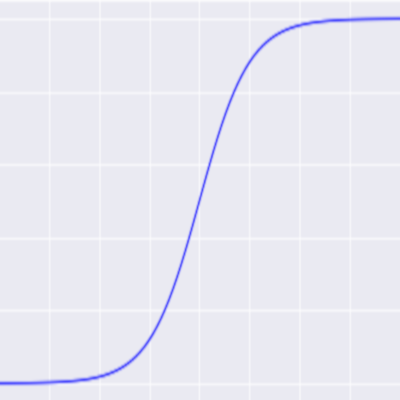## Cross-entropy classification

Introduction to the logistic and softmax classification functions and how to use them with the cross-entropy loss function.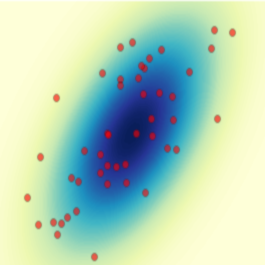## Multivariate normal distribution: a primer

Introduction to the multivariate normal distribution that will show you how to represent, visualize, sample, and compute conditionals from this distribution.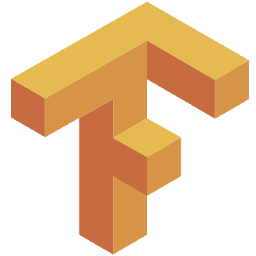## TensorFlow Estimator & Dataset APIs

How to use TensorFlow's Estimator and Dataset APIs to train models.